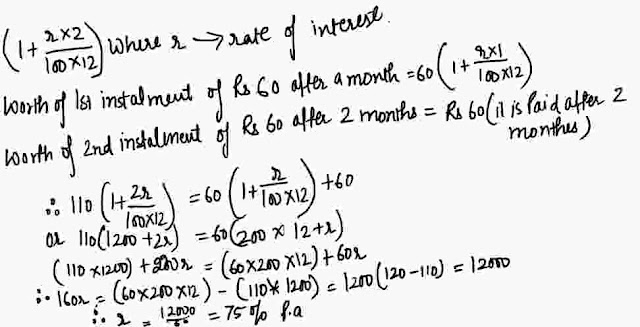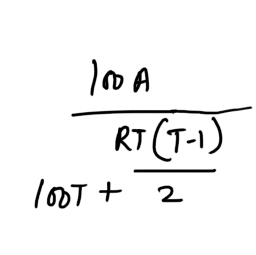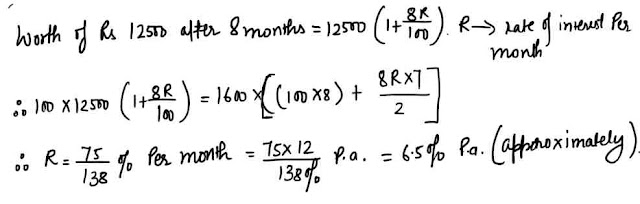New Students Offer - Use Code HELLO

# Instalments : 9 Important Questions for Upcoming Exams#### Ques 1.

A pressure cooker is available for Rs 180 cash or Rs 70 cash  down payment followed by Rs 60 a  month for 2 months . Find the rat e of interest charged under the instalment plan.
Ans 1. Present worth of the amount to be paid in instalments = rs (180-70) = 110
Worth of Rs 110 after 2 months  =#### Ques 2.

A house is  sold for Rs 30,000  cash or Rs 17,500 cash down payment  and  instalments of Rs  1,600 per month for eight months . Determine the rate of interest under the instalment plan.
Ans 2. It would  be quite tedious  to follow  the same manner of arriving at the answer  as is the preceding examples ( here there are 8 months ).
Instead let's apply a general formula for such problems (the formula  is derive the same approach  as is in the preceding  example) :
The annual instalment that will discharge  a debt of Rs A due in T years  at R%  p.a. simple interest isFor monthly  instalment the same formula  holds except for the fact that R% ' would be for  'per  month '.#### Ques 3.

I got a loan of Rs 3000 for the repair  of my house . The loan is to be paid back in 3 annual instalments . How much is each instalment if the interest  is compounded annually on the balance at 5% and is included in each isntalment ?
Ans 3. The loan is to be paid in 3 months instalments .
I will pay Rs ( 3000 ÷3) i.e. Rs 1000 every year together with the interest on the balance for 1 year.#### Ques 4.

A man borrows money on compound interest and return it in three annual instalments. Find the value of each instalment if the borrowed amount is Rs 12,610 and the rate of interest is 5% p.a.
Ans 4. Let each instalment  be Rs x.Ques 5.
A sun of Rs 6000 is to be paid in 3 annual  instalment .How much is each instalment if the interest is compounded annualy  on the 5% p.a. and it is to be  includfed in each instalment ?
(a) Rs 2800 , Rs 2700 , Rs 2600
(b) Rs 2300, Rs 2200, Rs 2100
(c) Rs 2000 , Rs 2000 , Rs 2000
(d) None of these
Ans 5. Rs 2300, Rs 2200, Rs 2100
Hint :  See solved example no. 4

#### Ques 6.

A house is sold for Rs 12000  cash  or Rs 7000 as cash down payment and Rs 630 a month for  8 months .Find the rate of interest per annum.
(a) 21.5%
(b) 2.15%
(c) 20%
(d) 23.25%
Ans 6. 2.15%

#### Ques 7.

A sum of Rs 2310 is to  be paid  in two equal annual instalment . How much is each instalment if the interest is compounded annually at 10%
(a) Rs 105
(b) Rs 1331
(c) Rs 1831
(d) Rs 813
Ans 7.  Rs 1331

#### Ques 8.

A sum of money borrowed  at 5%  per annum  compound interest is paid back in three equal annual installment . If each installment  is of Rs. 18,522 , the sum borrowed is
(a) Rs 15000
(b) Rs  10830
(c) Rs 50440
(d) Rs  81650
Ans 8.  Rs. 50440

#### Ques 9.

A sum of  money borrowed and  paid back in two equal annual instalments of Rs 882 each allowing  5% p.a. compound  interest . What is the sum  borrowed ?
(a) Rs 1640
(b) Rs  1050
(c) Rs 1380
(d) Rs 1180
Ans 9. Rs 1640